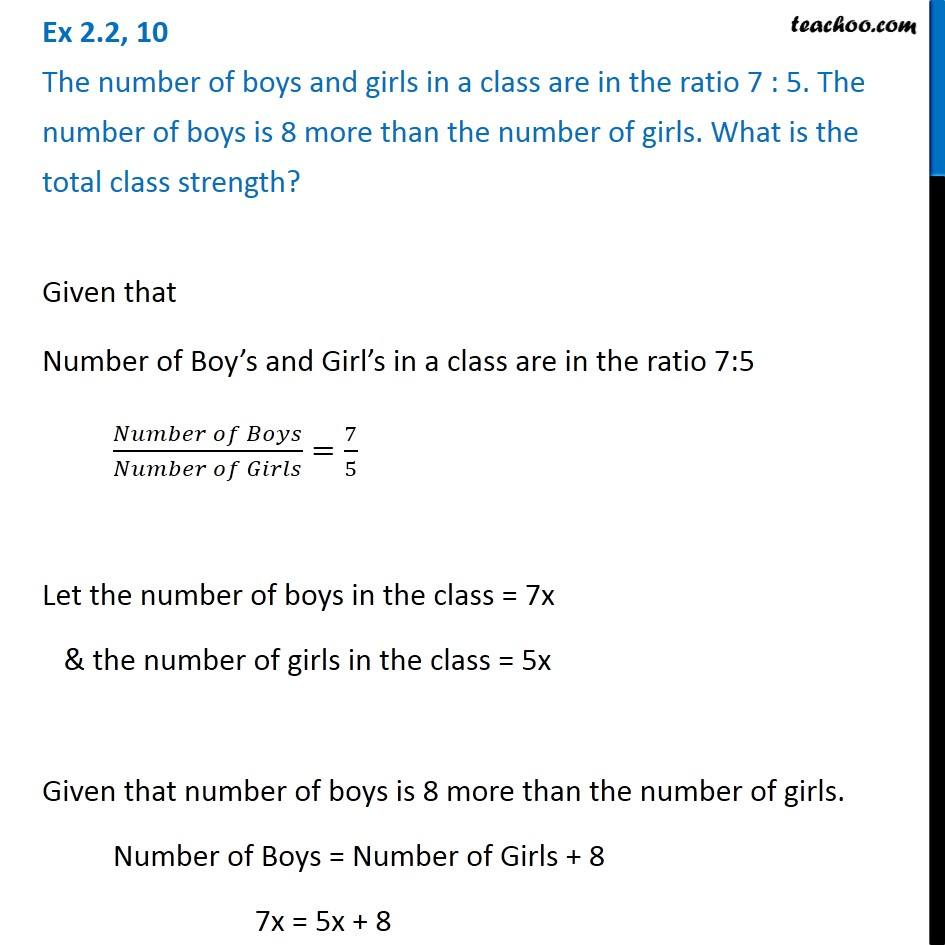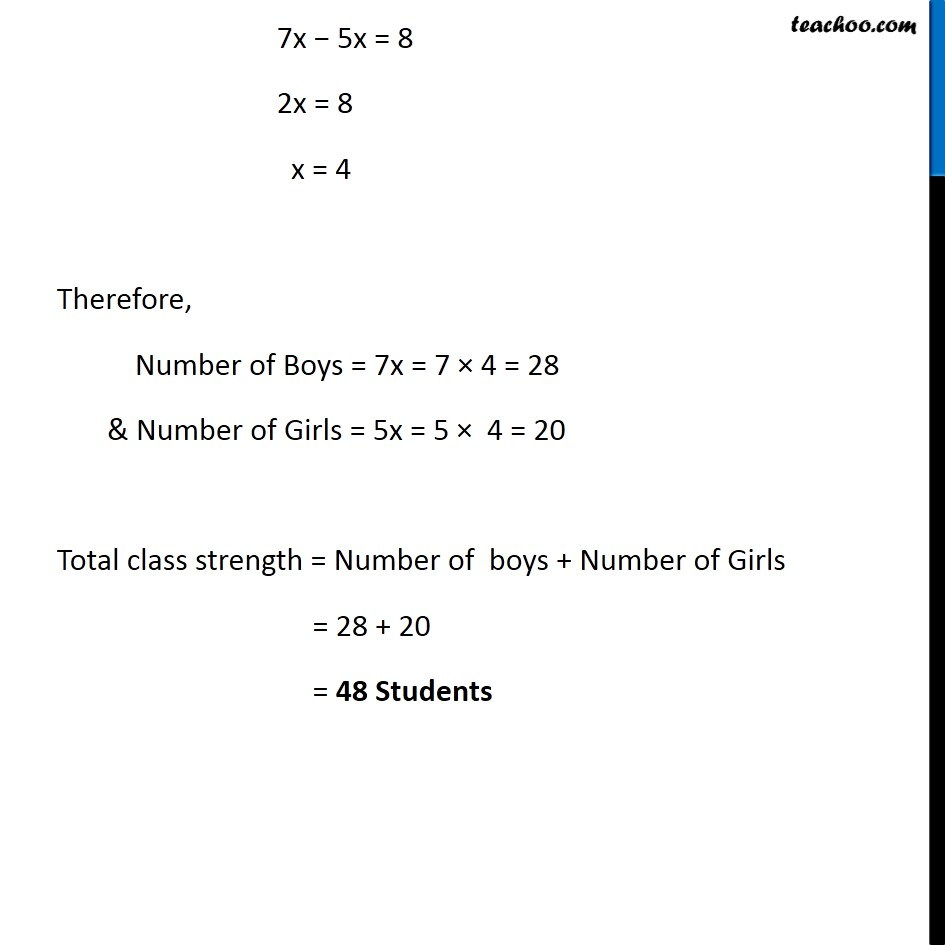Solving easy equations - Word Problems

Chapter 2 Class 8 Linear Equations in One Variable
Serial order wiseLearn in your speed, with individual attention - Teachoo Maths 1-on-1 Class

### Transcript

Question 10 The number of boys and girls in a class are in the ratio 7 : 5. The number of boys is 8 more than the number of girls. What is the total class strength?Given that Number of Boy’s and Girl’s in a class are in the ratio 7:5 (𝑁𝑢𝑚𝑏𝑒𝑟 𝑜𝑓 𝐵𝑜𝑦𝑠)/(𝑁𝑢𝑚𝑏𝑒𝑟 𝑜𝑓 𝐺𝑖𝑟𝑙𝑠)=7/5 Let the number of boys in the class = 7x & the number of girls in the class = 5x Given that number of boys is 8 more than the number of girls. Number of Boys = Number of Girls + 8 7x = 5x + 8 7x − 5x = 8 2x = 8 x = 4 Therefore, Number of Boys = 7x = 7 × 4 = 28 & Number of Girls = 5x = 5 × 4 = 20 Total class strength = Number of boys + Number of Girls = 28 + 20 = 48 Students